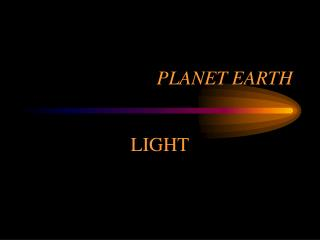DownloadDownload PresentationPLANET EARTH

# PLANET EARTH

Télécharger la présentation## PLANET EARTH

- - - - - - - - - - - - - - - - - - - - - - - - - - - E N D - - - - - - - - - - - - - - - - - - - - - - - - - - -
##### Presentation Transcript

1. PLANETEARTH LIGHT

2. Lab Experiments • Distance Behavior - I = A/r2 +B • Definition of color - The ratio of two colors at the same distance. • Color and Temperature - objects get more “blue” with increasing temperature. • Attenuation I = I0• e-•d

3. Source • Observations - visible • for Hydrogen • 1/ = R(1/22 - 1/n2) , R = 1.097 •107 m-1 • n = 3 red light  = 6562 •10-10 • n = 4 blue light  = 4861 •10-10 • This is an empirical formula! • Each atom has a unique spectrum! • Formulas like this, give the colors of light that come out of atoms.

4. Bohr Model • Quantized energy levels exist in all atoms. • For Hydrogen the energy of each level is • En = 13.6/n2 • Transitions between levels either absorb or release energy. Energy is released in the form of light packets or photons each of energy: • E = hc/ = hf Einstein postulates this for PE effect • the energy available is the difference between the two levels involved in the transition. • Is this consistent with the “observations” on the previous slide.

5. Bohr & Empirical • E2-n = 13.6 (1/22-1/n2) Bohr theory • hc/  = E2-n energy emitted as a photon • Then 1/  = 13.6/hc (1/22-1/n2) • Does 13.6/hc = R ?

6. Constants • R = 1.095x107 m-1 • h = 6.626x10-34 joule-sec • 1 ev = 1.6x10-19 joules • c = 3x108 m/sec